Electronic Devices and Amplifier Circuits with MATLAB Computing, Second Edition

# 3.5: Fixed Bias

## 3.5 Fixed Bias

The biasing method used in the transistor circuits in Figures 3.21 and 3.22 is known as fixed bias. Even though with modern technology transistors are components (parts) of integrated circuits, or ICs, some are used as single devices. To bias a transistor properly, one must establish a constant DC current in the emitter so that it will not be very sensitive to temperature variations and large variations in the value of ? among transistors of the same type. Also, the Q point must be chosen so that it will allow maximum signal swing from positive to negative values.

Figure 3.23 shows another configuration of an NPN transistor with fixed bias.Figure 3.23: NPN Transistor with fixed bias

Following the procedure of Example 3.8 we can simplify the circuit in Figure 3.23 with the use of Thevenin s theorem to the circuit in Figure 3.24.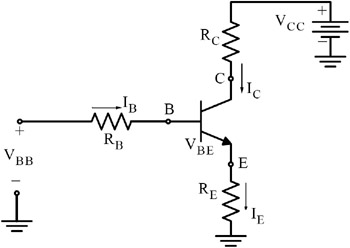Figure 3.24: The circuit in Figure 3.23 after application of Thevenin s theorem

With reference to Figures 3.23 and 3.24 we obtain the following relations: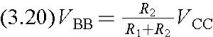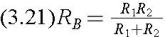It was stated earlier that it is imperative to keep variations in temperature and changes in ? values to a minimum and this can be achieved by making the emitter current I E fairly constant.

Therefore, let us now derive an expression for I E.

From Figure 3.24 with application of KVL we obtain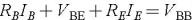and from Table 3.1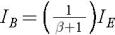Then,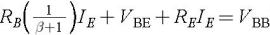or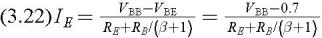From expression (3.22) we see that the emitter current I E will be fairly...

UNLIMITED FREE ACCESS TO THE WORLD'S BEST IDEAS